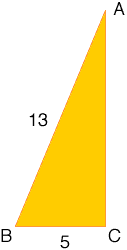Quandaries and Queries Name:  Kyle   Student, Secondary Question   I would like to know how to evaluate the problem of:  Arccos 5/13.   Thank you. Hi Kyle, I expect that what you want is some trig function of arccos(5/13), like maybe tan(arccos(5/13)) I would start by drawing a triangle.In this diagram the angle ABC has a cosine of 5/13. In other words arccos(5/13) = angle ABC By Pythagoras' theorem 52 + |CB|2 = 132 that is |CA|2 = 132 - 52 = 169 - 25 = 144 so |CA| = 12 Now you can read the trig functions of angle ABC from the diagram, for example tan(arccos(5/13)) = 12/5 I hope this helps,Penny Go to Math Central